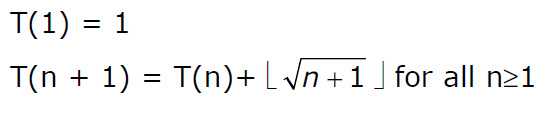# GATE | GATE-CS-2003 | Question 90

Consider the following recurrence relationThe value of T(m2) for m ≥ 1 is
(A) (m/6) (21m – 39) + 4
(B) (m/6) (4m2 – 3m + 5)
(C) (m/2) (m2.5 – 11m + 20) – 5
(D) (m/6) (5m3 – 34m2 + 137m – 104) + (5/6)

Explanation:

```One easy way to solve this is to try putting different
values of m.

For example, we know T(1) = 1. If we put m = 1, only A
and B satisfy the result.

m = 2

T(2) = T(1) + 1 = 2
T(3) = T(2) + 1 = 3
T(4) = T(3) + 2 = 5

Both A & B produce 5

m = 3
T(9) = T(4) + 2*5 + 1 = 5 + 10 + 1 = 16
Both A & B produce 16

m = 4
T(16) = T(9) + 3*7 + 1 = 16 + 21 + 1 = 38
Only B produces 38, A produces 34 which doesn't match ```
My Personal Notes arrow_drop_up
Article Tags :

Be the First to upvote.

Please write to us at contribute@geeksforgeeks.org to report any issue with the above content.